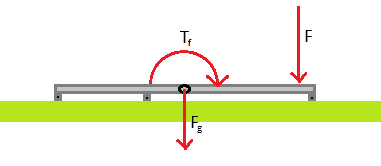# Applying force to a flat object with contact points

Hi all!

This isn't actually a homework question, but thought this subforum is the place for this anyway. It's been some ten years since I last touched the topic of mechanics and I guess this is a real simple question, but what can you do when I don't get it! Any help is appreciated!

How do I calculate the forces acting on contact points in situation like the picture?
There is the force from gravity on the object, and another force at some point pushing the object down. Torque in the pic is from the force pushing down on right.

Calculating torque from the force and lever arm is simple yes, but getting that back to forces on the contact points is what baffles me.## Answers and Replies

Pondering this a little further, does the moment of force need to be calculated relative to the contact points? I was under the impression that its calculated relative to mass center, but that seems to give me really counter intuitive results

SteamKing
Staff Emeritus
Science Advisor
Homework Helper
From your picture, it appears that there are three contact points. Without knowing something about the object, there are not enough equations of statics to calculate the reactions at each support knowing the loads and their locations relative to the supports.

SteamKing, thank you for you reply. I should have said that I would love to know how to calculate these things, not the exact numeric results.

How to, meaning the equations. Or an explanation how to calculate the resulting force, I can look the equations up from wikipedia.

First I thought calculating the forces would be:
First calculate the momentum relative to center of mass ( M = F*l, M = momentum, F = force, l = lever arm length) and then reverse it for each point of contact to get the force acting on that point. But that leads me nowhere, for example if I would have a contact point close to CM the force would be huge as the divider is small. F = M/l, l = 0.001m -> huge numbers.

Then it came to my mind that maybe the moment should be calculated relative to every contact point and from those (in this case, 3) moments calculate the forces on each contact point. Is this the correct way?

haruspex
Science Advisor
Homework Helper
Gold Member
2020 Award
You will only get two equations from a set-up like this, one for linear vertical forces and one for moments. You can take moments about various points, but it does not give you any extra equations: given any two you can deduce the rest.
Therefore, you can only solve it in general for two contact points. With more, there are multiple combinations of forces that satisfy the equations. To get further you have to make some assumptions about the nature of the surfaces, typically, that they are uniformly springy (how ever slightly). So if the two planes are perfectly flat and make some small angle, you can calculate the ratios of the forces at the contact points.